# Boolean Algebra Digital Electronics What is Boolean Algebra

• Slides: 19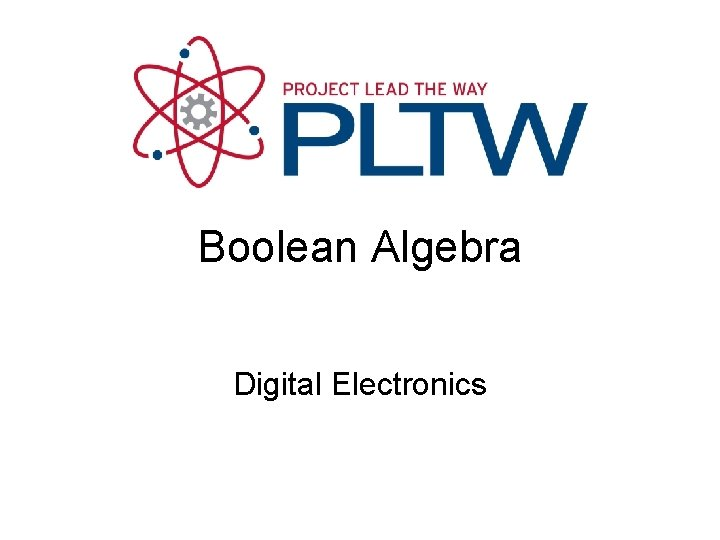Boolean Algebra Digital Electronics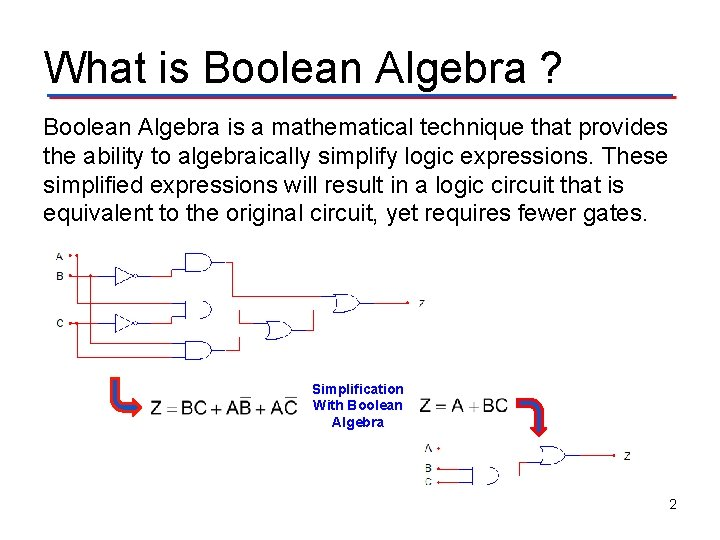What is Boolean Algebra ? Boolean Algebra is a mathematical technique that provides the ability to algebraically simplify logic expressions. These simplified expressions will result in a logic circuit that is equivalent to the original circuit, yet requires fewer gates. Simplification With Boolean Algebra 2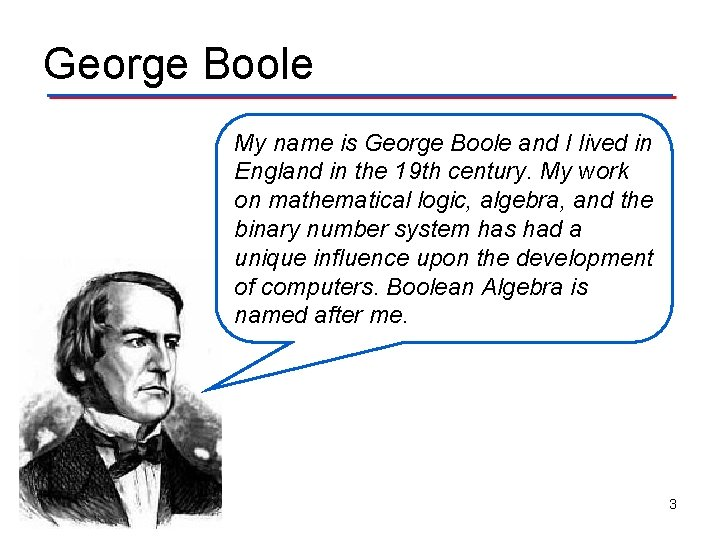George Boole My name is George Boole and I lived in England in the 19 th century. My work on mathematical logic, algebra, and the binary number system has had a unique influence upon the development of computers. Boolean Algebra is named after me. 3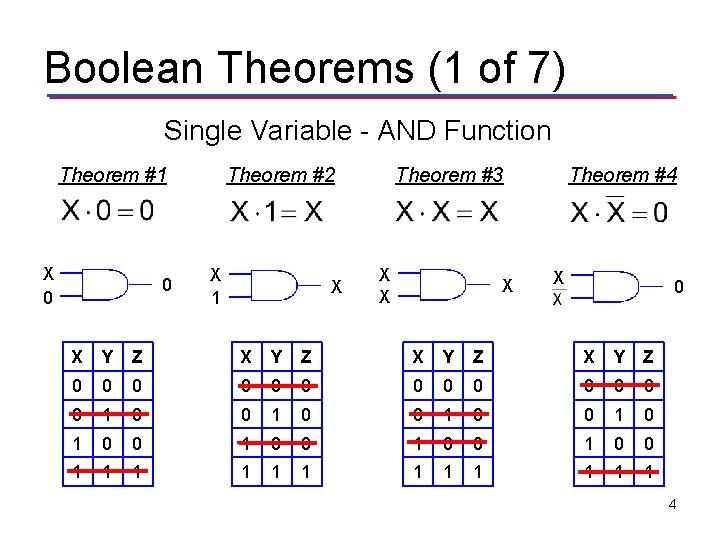Boolean Theorems (1 of 7) Single Variable - AND Function Theorem #1 X 0 0 Theorem #2 X 1 X Theorem #3 X X X Theorem #4 X 0 X Y Z 0 0 0 0 1 0 0 1 0 0 1 1 1 4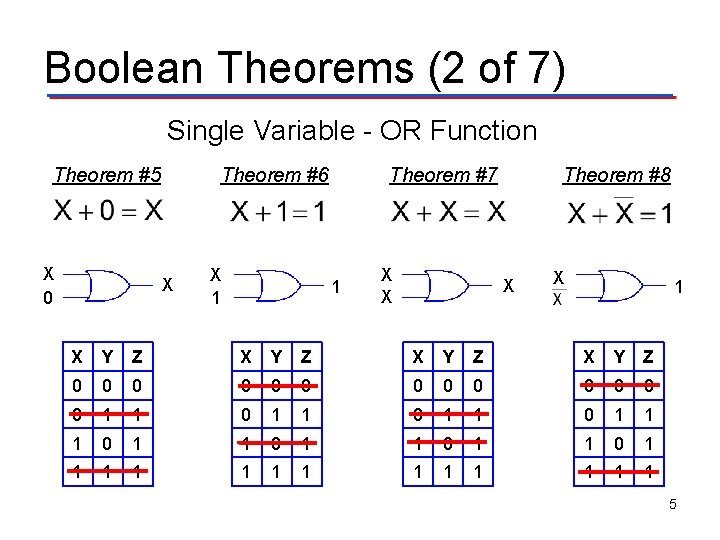Boolean Theorems (2 of 7) Single Variable - OR Function Theorem #5 X 0 Theorem #6 X X 1 Theorem #7 1 X X Theorem #8 X X 1 X Y Z 0 0 0 0 1 1 0 1 1 1 1 1 5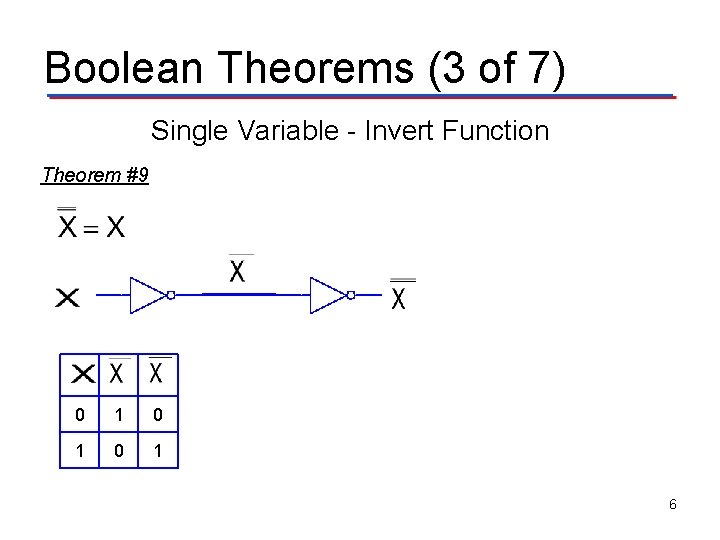Boolean Theorems (3 of 7) Single Variable - Invert Function Theorem #9 0 1 0 1 6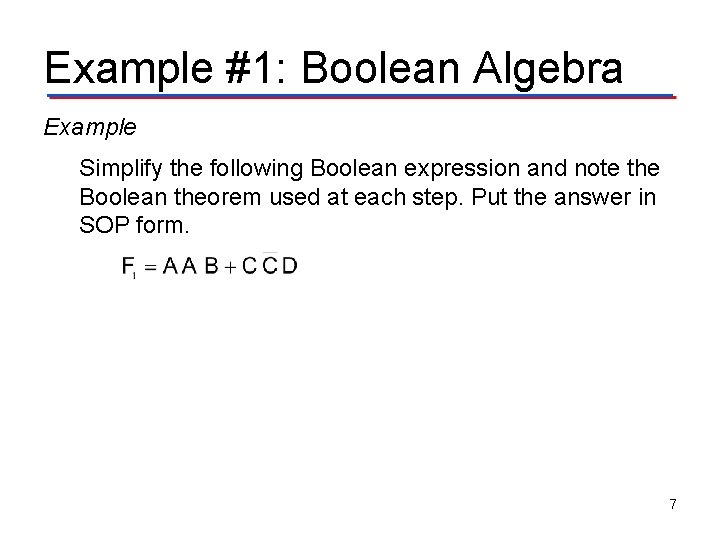Example #1: Boolean Algebra Example Simplify the following Boolean expression and note the Boolean theorem used at each step. Put the answer in SOP form. 7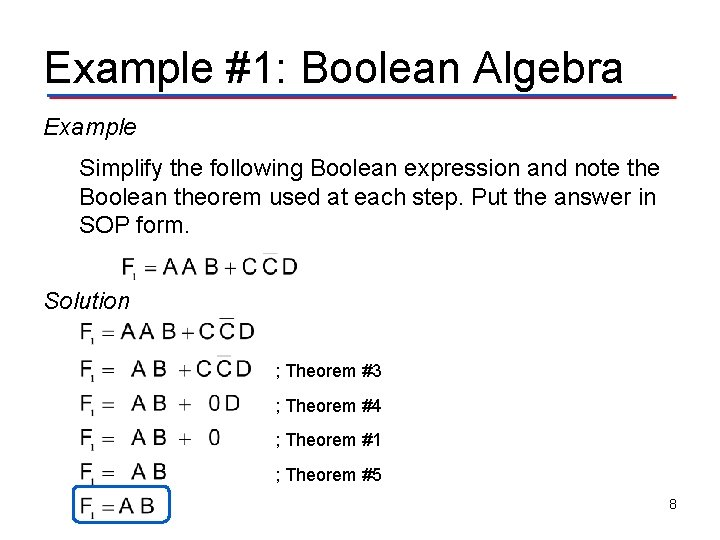Example #1: Boolean Algebra Example Simplify the following Boolean expression and note the Boolean theorem used at each step. Put the answer in SOP form. Solution ; Theorem #3 ; Theorem #4 ; Theorem #1 ; Theorem #5 8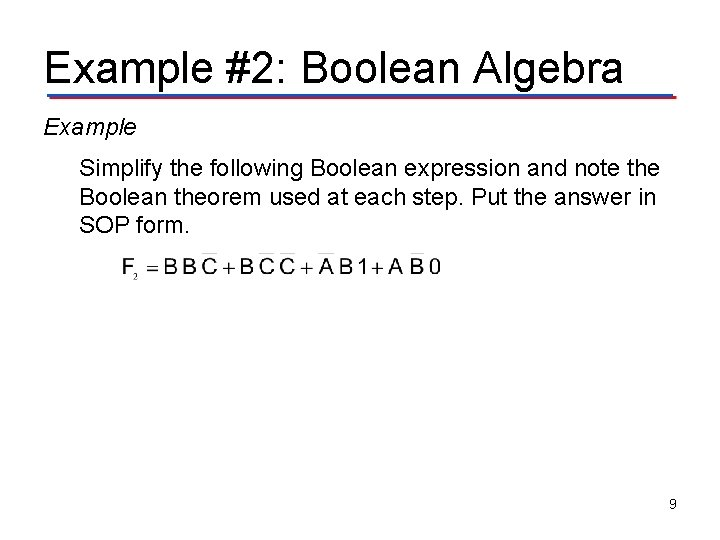Example #2: Boolean Algebra Example Simplify the following Boolean expression and note the Boolean theorem used at each step. Put the answer in SOP form. 9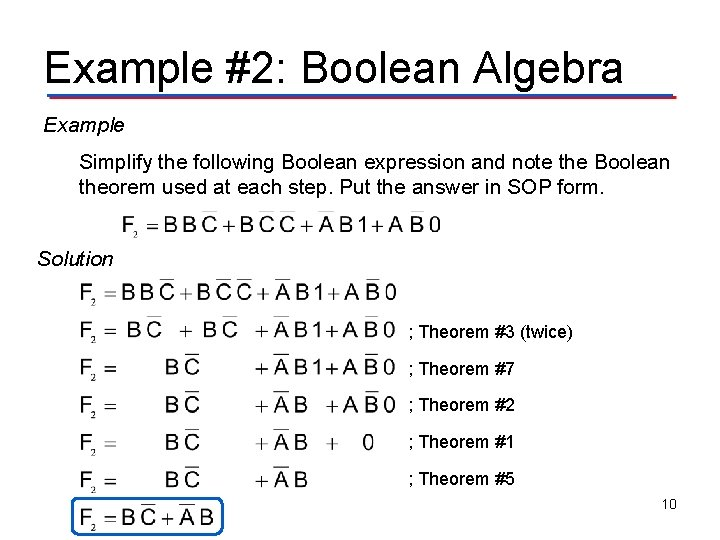Example #2: Boolean Algebra Example Simplify the following Boolean expression and note the Boolean theorem used at each step. Put the answer in SOP form. Solution ; Theorem #3 (twice) ; Theorem #7 ; Theorem #2 ; Theorem #1 ; Theorem #5 10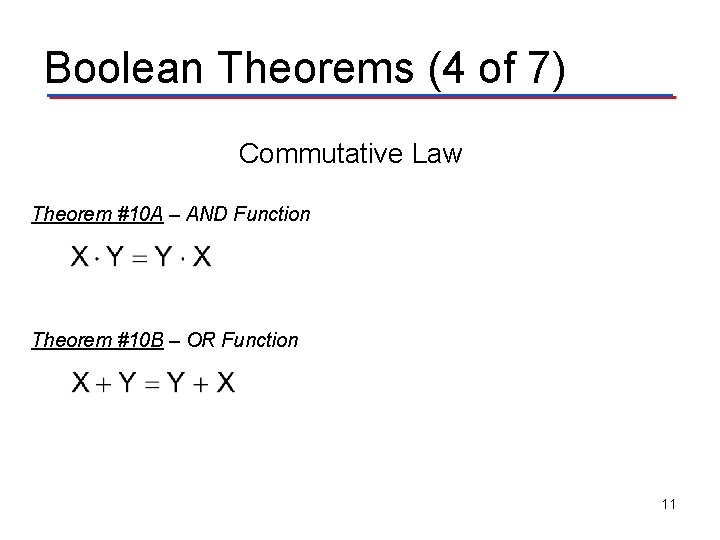Boolean Theorems (4 of 7) Commutative Law Theorem #10 A – AND Function Theorem #10 B – OR Function 11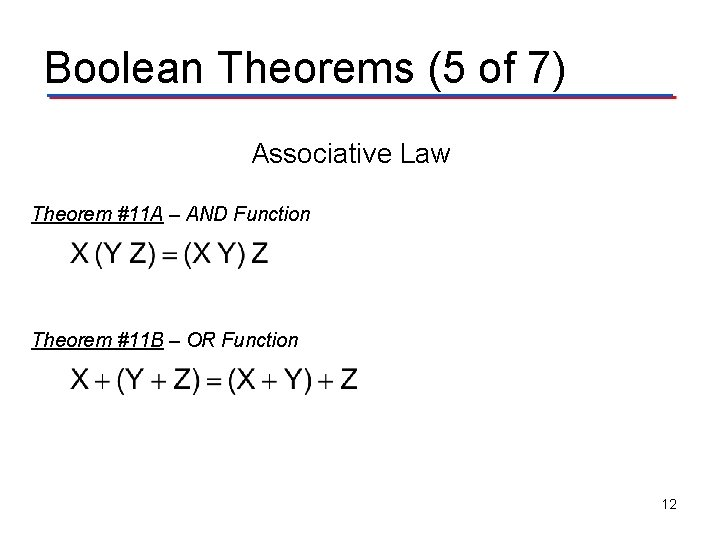Boolean Theorems (5 of 7) Associative Law Theorem #11 A – AND Function Theorem #11 B – OR Function 12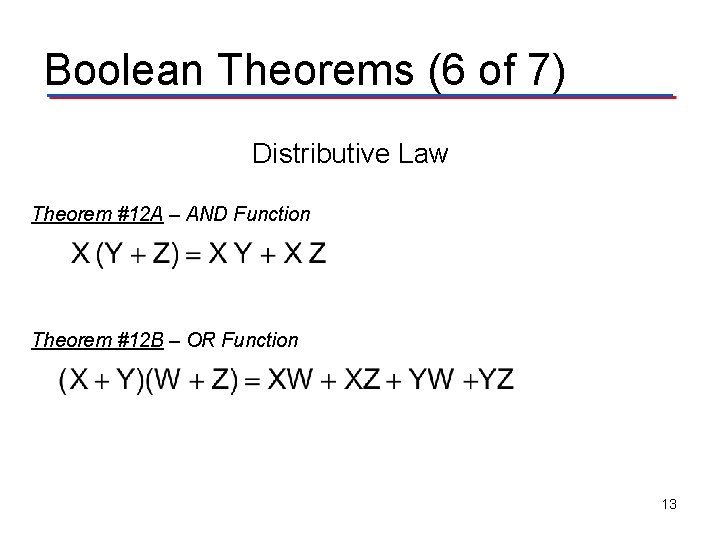Boolean Theorems (6 of 7) Distributive Law Theorem #12 A – AND Function Theorem #12 B – OR Function 13Example #3: Boolean Algebra Example Simplify the following Boolean expression and note the Boolean theorem used at each step. Put the answer in SOP form. 14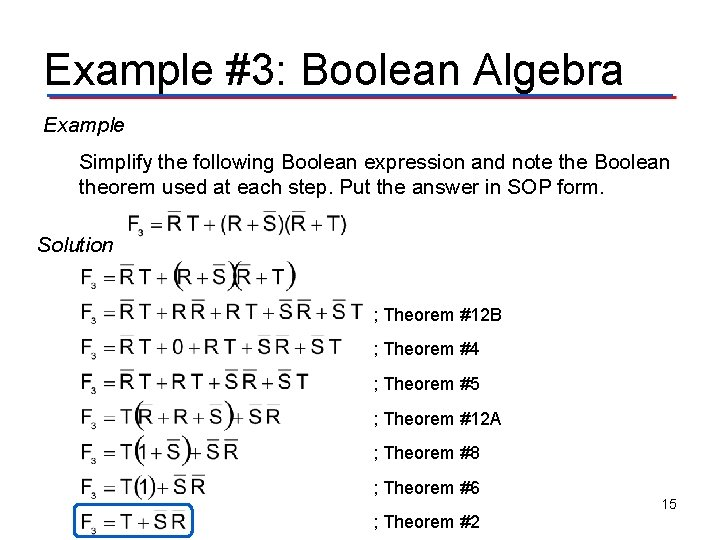Example #3: Boolean Algebra Example Simplify the following Boolean expression and note the Boolean theorem used at each step. Put the answer in SOP form. Solution ; Theorem #12 B ; Theorem #4 ; Theorem #5 ; Theorem #12 A ; Theorem #8 ; Theorem #6 ; Theorem #2 15Boolean Theorems (7 of 7) Consensus Theorem #13 A Theorem #13 C Theorem #13 B Theorem #13 D 16Example #4: Boolean Algebra Example Simplify the following Boolean expression and note the Boolean theorem used at each step. Put the answer in SOP form. 17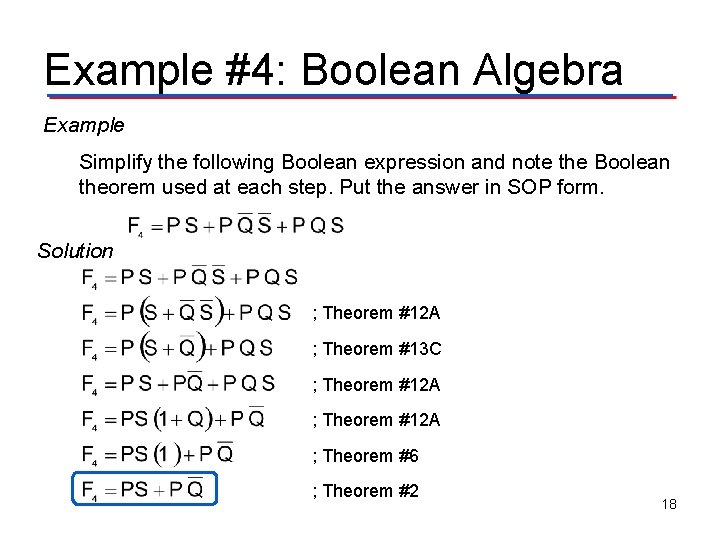Example #4: Boolean Algebra Example Simplify the following Boolean expression and note the Boolean theorem used at each step. Put the answer in SOP form. Solution ; Theorem #12 A ; Theorem #13 C ; Theorem #12 A ; Theorem #6 ; Theorem #2 18Summary Boolean Theorems Commutative Law Associative Law Distributive Law Consensus Theorem 19